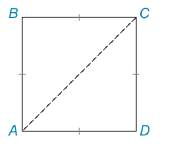Chapter 3.5, Problem 34E### Elementary Geometry for College St...

6th Edition
Daniel C. Alexander + 1 other
ISBN: 9781285195698

#### Solutions

Chapter
Section### Elementary Geometry for College St...

6th Edition
Daniel C. Alexander + 1 other
ISBN: 9781285195698
Textbook Problem
6 views

# In Exercises 31 to 34, apply a form of Theorem 3.5.10. Prove by the indirect method: “The length of a diagonal of a square is not equal in length to the length of any of the sides of the square.”To determine

To prove:

The length of a diagonal of a square is not equal in length to the length of any of the sides of the square.

Explanation

Given:

The square ABCD is shown in the below figure,

Figure (1)

Approach:

The length of any side of a triangle must lie between the sum and difference of the lengths of the other two sides.

Assume, the length of all the sides of the square is x and the length of the diagonal of the square is y.

Consider the triangle ΔABC,

The length of the two sides of the triangle ΔABC is x

### Still sussing out bartleby?

Check out a sample textbook solution.

See a sample solution

#### The Solution to Your Study Problems

Bartleby provides explanations to thousands of textbook problems written by our experts, many with advanced degrees!

Get Started

#### Find more solutions based on key concepts